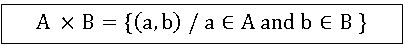1. Functoins

Set: A collection of well-defined objects is called set. Ordered pair: Two elements a and b listed in a specific order form. An ordered pair denoted by (a, b). Cartesian product: Let A and B are two non- empty sets. The Cartesian product of A and B is denoted by A × B and is defined as set of all ordered pairs (a, b) where a ϵ A and b ϵBRel